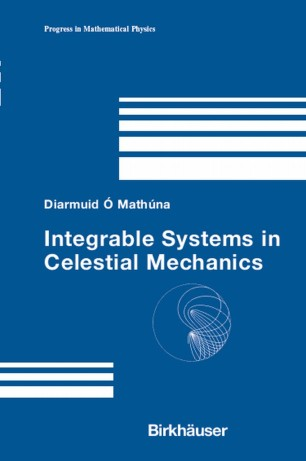# Integrable Systems in Celestial Mechanics

• Authors
• Diarmuid Ó MathúnaBook

Part of the Progress in Mathematical Physics book series (PMP, volume 51)

1. Front Matter
Pages 1-10
2. Pages 1-19
3. Pages 21-27
4. Pages 29-48
5. Pages 49-111
6. Pages 113-142
7. Pages 143-192
8. Pages 193-210
9. Back Matter
Pages 1-24

### Introduction

This work presents a unified treatment of three important integrable problems relevant to both Celestial and Quantum Mechanics. Under discussion are the Kepler (two-body) problem and the Euler (two-fixed center) problem, the latter being the more complex and more instructive, as it exhibits a richer and more varied solution structure. Further, because of the interesting investigations by the 20th century mathematical physicist J.P. Vinti, the Euler problem is now recognized as being intimately linked to the Vinti (Earth-satellite) problem.

Here the analysis of these problems is shown to follow a definite shared pattern yielding exact forms for the solutions. A central feature is the detailed treatment of the planar Euler problem where the solutions are expressed in terms of Jacobian elliptic functions, yielding analytic representations for the orbits over the entire parameter range. This exhibits the rich and varied solution patterns that emerge in the Euler problem, which are illustrated in the appendix. A comparably detailed analysis is performed for the Earth-satellite (Vinti) problem.

Key features:

* Highlights shared features in the unified treatment of the Kepler, Euler, and Vinti problems

* Raises challenges in analysis and astronomy, placing this trio of problems in the modern context

* Includes a full analysis of the planar Euler problem

* Highlights the complex and surprising orbit patterns that arise from the Euler problem

* Provides a detailed analysis and solution for the Earth-satellite problem

The analysis and results in this work will be of interest to graduate students in mathematics and physics (including physical chemistry) and researchers concerned with the general areas of dynamical systems, statistical mechanics, and mathematical physics and has direct application to celestial mechanics, astronomy, orbital mechanics, and aerospace engineering.

### Keywords

Euler problem Kepler problem Lagrangian mechanics Potential Vinti problem calculus differential equation earth-satellite problem mathematical physics two-body problem two-fixed center problem